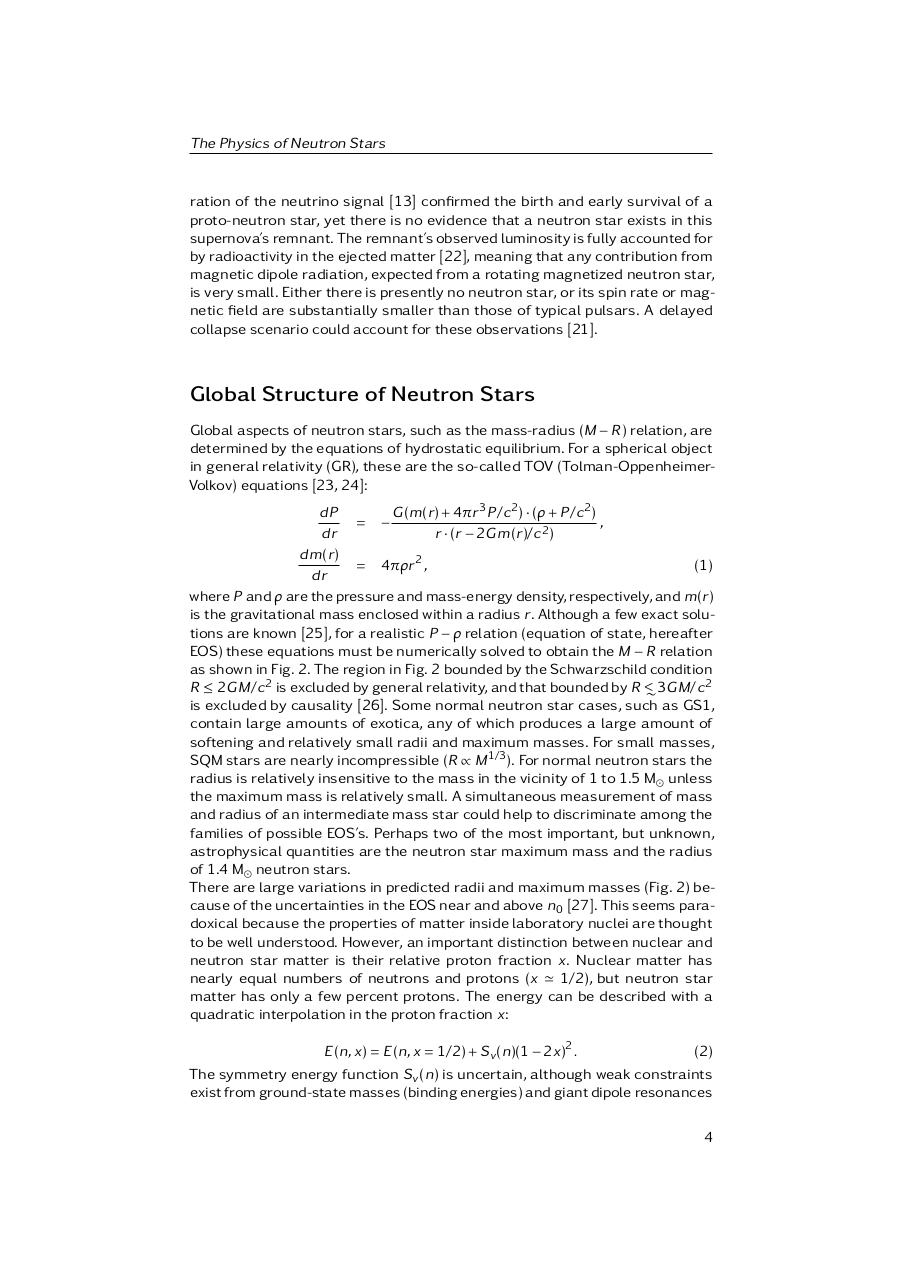# J.M. Lattimer and M. Prakash. The Physics of Neutron Stars.pdfPage 1 2 3 45619

#### Text preview

The Physics of Neutron Stars

ration of the neutrino signal  confirmed the birth and early survival of a
proto-neutron star, yet there is no evidence that a neutron star exists in this
supernova’s remnant. The remnant’s observed luminosity is fully accounted for
by radioactivity in the ejected matter , meaning that any contribution from
magnetic dipole radiation, expected from a rotating magnetized neutron star,
is very small. Either there is presently no neutron star, or its spin rate or magnetic field are substantially smaller than those of typical pulsars. A delayed
collapse scenario could account for these observations .

Global Structure of Neutron Stars
Global aspects of neutron stars, such as the mass-radius (M − R) relation, are
determined by the equations of hydrostatic equilibrium. For a spherical object
in general relativity (GR), these are the so-called TOV (Tolman-OppenheimerVolkov) equations [23, 24]:
G (m(r) + 4ár 3 P/c 2 ) · (â + P/c 2 )
dP
= −
,
dr
r · (r − 2G m(r)/c 2 )
d m(r)
= 4áâr 2 ,
(1)
dr
where P and â are the pressure and mass-energy density, respectively, and m(r)
is the gravitational mass enclosed within a radius r. Although a few exact solutions are known , for a realistic P − â relation (equation of state, hereafter
EOS) these equations must be numerically solved to obtain the M − R relation
as shown in Fig. 2. The region in Fig. 2 bounded by the Schwarzschild condition
2
R ≤ 2G M/c 2 is excluded by general relativity, and that bounded by R &lt;
∼ 3G M/c
is excluded by causality . Some normal neutron star cases, such as GS1,
contain large amounts of exotica, any of which produces a large amount of
softening and relatively small radii and maximum masses. For small masses,
SQM stars are nearly incompressible (R ∝ M 1/3 ). For normal neutron stars the
radius is relatively insensitive to the mass in the vicinity of 1 to 1.5 M unless
the maximum mass is relatively small. A simultaneous measurement of mass
and radius of an intermediate mass star could help to discriminate among the
families of possible EOS’s. Perhaps two of the most important, but unknown,
astrophysical quantities are the neutron star maximum mass and the radius
of 1.4 M neutron stars.
There are large variations in predicted radii and maximum masses (Fig. 2) because of the uncertainties in the EOS near and above n0 . This seems paradoxical because the properties of matter inside laboratory nuclei are thought
to be well understood. However, an important distinction between nuclear and
neutron star matter is their relative proton fraction x. Nuclear matter has
nearly equal numbers of neutrons and protons (x ' 1/2), but neutron star
matter has only a few percent protons. The energy can be described with a
quadratic interpolation in the proton fraction x:
E(n, x) = E(n, x = 1/2) + S v (n)(1 − 2x)2 .

(2)

The symmetry energy function S v (n) is uncertain, although weak constraints
exist from ground-state masses (binding energies) and giant dipole resonances
4